FIN415 Class Web Page, Spring '16

Jacksonville University

Instructor: Maggie Foley

Term Project Part I
Term project part II  (excel question)

Weekly SCHEDULE, LINKS, FILES and Questions

Week

Coverage, HW, Supplements

-        Required

Videos (optional)

Week 1 –

Mar 4

Part I: Review of the global economy

Capital Flow Chart

Is Fed powerful?

Global Outlook for Growth of Gross Domestic Product, 2014-2025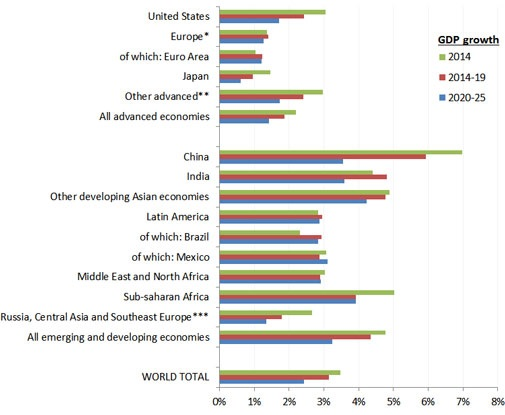Distribution of World GDP in 2000, 2012 and 2025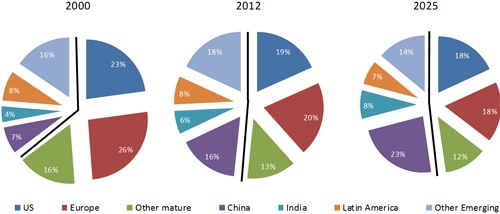Part II: Chapter 2  International Flow of Funds

Chapter 2 (PPT)

Requirements:

·         What is BOP? What is current account?

Balance of payments (BoP) accounts are an accounting record of all monetary transactions between a country and the rest of the world. These transactions include payments for the country's export and imports of goods, services, financial capital, and financial transfers.

A country's current account is one of the two components of its balance of payments, the other being the capital account. The current account consists of the balance of trade, net factor income (earnings on foreign investments minus payments made to foreign investors) and cash transfers

A current account surplus increases a country's net foreign assets by the corresponding amount, and a current account deficit does the reverse.

A country's balance of trade is the net or difference between the country's export of goods and services and its imports of goods and services, ignoring all financial transfers, investments and other components. A country is said to have a trade surplus if its exports exceed its imports, and a trade deficit if its imports exceed its exports.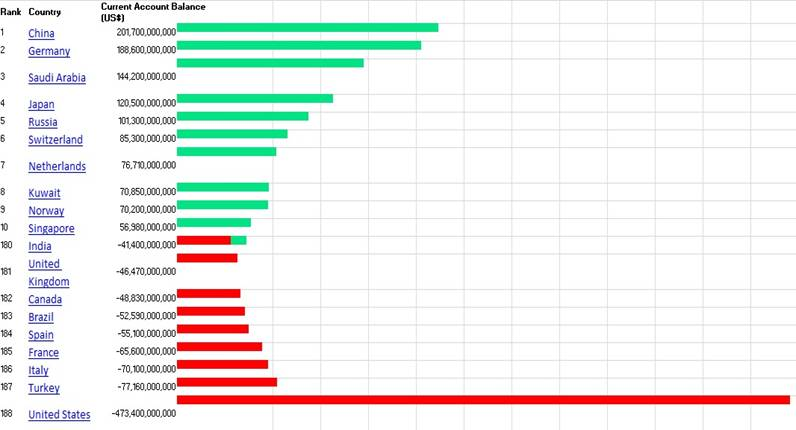Source: indexmundi.com and world bank

·         Can US devaluate currency to eliminate current account deficit?

1.      \$ devalued, then export become cheaper and increased; imports more expensive and decreased; seems like current account deficit could disappear.

2.      However, in short run, demand is not so flexible and thereby current account might get worsen.

3.      Demand will improve with time, so current account improves with time, but not immediately.

4.      \$ depreciated, it becomes less attractive to foreign government and investors. The borrowing cost to cover the deficit will increase.

5.      \$ depreciated, living standard might drop.

6.      Competitor can drop price; Countries can devalue their currencies.

·         Evolution of international monetary system

1.      Bimetallism: Before 1875

2.      Classical Gold Standard: 1875-1914

International value of currency was determined by its fixed relationship to gold.

Gold was used to settle international accounts, so the risk of trading with other countries could be reduced.

3.      Interwar Period: 1915-1944

Countries suspended gold standard during the WWI, to increase money supply and pay for the war.

Countries relied on a partial gold standard and partly other countries’ currencies during the WWII

4.      Bretton Woods System: 1945-1972

All currencies were pegged to US\$.

US\$ was the only currency that was backed by gold.

US\$ was world currency at that time.

5.      The Flexible Exchange Rate Regime: 1973-Present

Exercise:

If the dollar is pegged to gold at U.S. \$30 = 1 ounce of gold, and the British pound is pegged to gold at £6 = 1 ounce of gold. What should be the exchange rate between U.S. \$ and British £? What will happen if this exchange rate does not hold, such as £1 = \$4?

·         What is European union? What is Euro zone? Who is ECB? You think EU will collapse? What about British exit from EU?

EU: free movement of goods, people, services, and capital.

EU: an economic and political union of 28 member states in Europe.

Euro zone: or euro area, is an economic and monetary union of 18 EU member states that have adopted euro.

ECB: in charge of monetary policy of euro zone.

Video: The European Debt Crisis Visualized (well explained)

HW – Chapter 2

1.      If the dollar is pegged to gold at U.S. \$30 = 1 ounce of gold, and the British pound is pegged to gold at £6 = 1 ounce of gold. What should be the exchange rate between U.S. \$ and British £?

How much can you make without any risk, if this exchange rate is £1 = \$6? Assume that your initial investment is \$1,200.

2.      Fed is in charge of monetary policy. How? What is opinion about Fed?

For reference: WSJ papers here

4.      Financial crisis started from bad loans. Do you support the bailout of banks by US government? Why or why not?

5. Internet exercises (not required, for interested students only)

a.       IMF, world bank and UN are only a few of the major organizations that track, report  and aid international economic and financial development. Using these website, you can summarize the economic outlook for each country.

World bank: www.worldbank.org

Bank of international settlement: www.bis.org/index.htm

b.      St. Louis Federal Reserve provides a large amount of recent open economy macroeconomic data online. You can track down BOP and GDP data for the major industrial countries.

Recent international economic data: research.stlouisfed.org/publications

Balance of Payments statistics: research.stlouisfed.org/fred2/categories/125

Reference of useful websites for global economy

Current Account (BOP) Data – World Bank

IMF, world bank and UN are only a few of the major organizations that track, report  and aid international economic and financial development. Using these website, you can summarize the economic outlook for each country.

World bank: www.worldbank.org

Bank of international settlement:  www.bis.org/index.htm

St. Louis Federal Reserve provides a large amount of recent open economy macroeconomic data online. You can track down BOP and GDP data for the major industrial countries.

Recent international economic data: research.stlouisfed.org/publicaitons/iet

**  Euro Crisis  for reference***

ECB Time Line of Financial Crisis

The great Euro crisis 2012

Video: The European Debt Crisis Visualized (well explained)

Week 2, 3

Reference:

Go to www.forex.com and set up a practice account and you can trade with \$50,000 virtue money.

Visit http://www.dailyfx.com/to get daily foreign exchange market news.

Requirements:

·         US is using floating exchange rate system. What is the advantage and disadvantage of this system? Did US government intervene the foreign exchange market?

·         Chinese currency is pegged to US\$. What is the advantage and disadvantage of this system? What about let it float, instead of holding its value at a fixed rate?

·         Who are the major players in the FX market?

·         What is direct and indirect quote and cross rate

1.      Direct quote is the local currency price of one unit of foreign currency. e.g. In New York City \$1.38/.

2.      Indirect quote is the inverse of the direct quote. It is the quotations that represent the number of units of a foreign currency per dollar. e.g. In New York City 0.72/\$.

3.      Cross Exchange Rates: it is the quotations that represent the number of units of a foreign currency per another foreign currency. e.g. In New York City: 0.90/£.

4.      You can get the cross exchange rates at www.forex.com

### The Forex bid is the price at which you can sell a certain Forex currency pair for (for base currency)..

Bid price represents what will be obtained in the quote currency when selling one unit of the base currency.

The ask price represents what has to be paid in the quote currency to obtain one unit of the base currency.

For instance, EUR/USD then EUR is the base and USD is the quote currency.

The bid price is seen on the left side of the Forex quote. For example, for the EUR/USD quote 1.2728/31, the bid price is 1.2728. This means you can sell one Euro for \$1.2728. The ask price is 1.2731. It means you can buy 1 Euro for \$1.2731.

Exercise:

Assume you have \$1000 and bid rate is \$1.52/£ and ask rate is \$1.60 /£. Meanwhile, the bid rate is quoted as 0.625 £/\$ and the ask rate is quoted as 0.6579 £/\$.  If you convert it to £ and then convert it back to \$, what will happen?

Answer: Sell at bid and buy at ask price (ask is always higher than bid so you buy high and sell low, since you are dealing with the bank). So here with \$1000, you sell at bid 0.625 £/\$ so you get 625£

(\$1000* 0.625 £/\$ = 625£). With 625£, you sell at bid \$1.52/£, so you get \$950 (625£ * \$1.52/£ = \$950)

Or

you buy at ask price. So here with \$1000, you want to buy £ @ 0.6579 £/\$,  so you get 657.9£

(\$1000* 0.6579 £/\$ = 657.9£). With 657.9£, you buy \$ at ask \$1.60/£, so you get \$950 (657.9£ * \$1.60/£ = \$950)

Note that the two sets of quotes are just inverse of each other. So it does not matter which set you are using.

HOMEWORK of CHAPTER 3

Compute the bid/ask percentage spread for Mexican peso retail transactions in which the ask rate is \$.11 and the bid rate is \$.10

2. Indirect Exchange Rate

If the direct exchange rate of the euro is worth \$1.25, what is the indirect rate of the euro? That is, what is the value of a dollar in euros?

3. Cross Exchange Rate

Assume Poland currency (the zloty) is worth \$.17 and the Japanese yen is worth \$.008. What is the cross rate of the zloty with respect to yen? That is, how many yen equal a zloty?

4. Foreign Exchange

You just came back from Canada, where the Canadian dollar was worth \$.70.

You still have C\$200 from your trip and could exchange them for dollars at the airport, but the airport foreign exchange desk will only buy them for \$.60. Next week, you will be going to Mexico and will need pesos. The airport foreign exchange desk will sell you pesos for \$.10 per peso. You met a tourist at the airport who is from Mexico and is on his way to Canada. He is willing to buy your C\$200 for 1,300 pesos. Should you accept the offer or cash the Canadian dollars in at the airport? Explain.

5.      Do you anticipate China to adopt floating exchange system? Why or why not? Do you expect US to go back to fixed exchange rate system? Why or why not?

Chapter 4: Determinants of foreign exchange rate

Currency value is determined by demand and supply, if not under control by the government.

Think about the changes in demand and supply when the following changes occur.

·         Inflation goes up (or down)

·         Real interest rate goes up (or down)

·         Domestic residents’ income goes up (or down)

·         Current account goes up (or down)

·         Public debt goes up (or down)

·         Recession or crisis

·         Other accidental events

Examples – Video

http://www.investopedia.com/video/play/main-factors-influence-exchange-rates/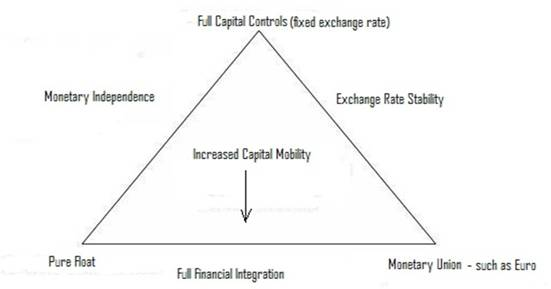The impossible trinity

A country cannot be on all three sides of the triangle at once.

It must give up one of the three attributes if it is to achieve one of the states described by the corner of the triangle.

Assume that world interest rate is at 5%. If the home central bank tries to set domestic interest rate at a rate lower than 5%, for example at 2%, there will be a depreciation pressure on the home currency, because investors would want to sell their low yielding domestic currency and buy higher yielding foreign currency. If the central bank also wants to have free capital flows, the only way the central bank could prevent depreciation of the home currency is to sell its foreign currency reserves. Since foreign currency reserves of a central bank is limited, once the reserves are depleted, the domestic currency will depreciate.

Hence, the all three of the policy objectives mentioned above cannot be pursued simultaneously. A central bank has to forgo one of the three objectives. Therefore a central bank has three policy combination options.

a) Fixed Exchange Rate and Free Capital Flow (pure float) – monetary independence

b) Independent Monetary Policy and Free Capital Flow (pure float) – full financial integration

c) Fixed Exchange Rate and Independent Monetary Policy – exchange rate stability

Class discussion: find a country that fits the following regimes and then discuss for the rational of that country’s adoption of the system based on the “impossible trinity”.

Exchange Rate Regimes from http://www.imf.org/external/np/mfd/er/2004/eng/0604.htm (IMF)

Class Exercise1: Chicago bank expects the exchange rate of the NZ\$ to appreciate from \$0.50 to \$0.52 in 30 days.

Chicago bank can borrow \$20m on a short term basis.

Currency                     Lending Rate              Borrowing rate

\$                              6.72%                          7.20%

NZ\$             6.48%                          6.96%

Question: If Chicago bank anticipate NZ\$ to appreciate, how shall it trade? (refer to ppt)

NZ\$ will appreciate, so you should buy NZ\$ now and sell later. Borrow \$à convert to NZ\$ today à lend it for 30 days à convert to \$ 30 days later àpayback the \$ loan.

Convert the borrowed \$ to NZ\$ today. So your NZ\$ worth: \$20m / 0.50 \$/NZ\$=40m NZ\$.

Lend NZ\$ for 6.48% * 30/360=0.54% and get

40m NZ\$ *(1+0.54%)=40,216,000 NZ\$ 30 days later.

Your borrowed \$20m should be paid back for

20m *(1+7.2%* 30/360)=\$20.12m. This equals to \$20.12m / 0.52 \$/NZ\$=NZ\$ 38,692,308.

So the profit is:

40,206000-38,692,308 = NZ\$1,523,692.

Your \$ profit is: NZ\$1,523,692 *0.52\$/NZ\$=\$792,320.

Class Exercise 2: Blue Demon Bank expects that the Mexican peso will depreciate against the dollar from its spot rate of \$.15 to \$.14 in 10 days. The following interbank lending and borrowing rates exist:

Lending Rate Borrowing Rate

U.S. dollar       8.0%    8.3%

Mexican peso  8.5%    8.7%

Assume that Blue Demon Bank has a borrowing capacity of either \$10 million or 70 million pesos in the interbank market, depending on which currency it wants to borrow.

a.                   How could Blue Demon Bank attempt to capitalize on its expectations without using deposited funds? Estimate the profits that could be generated from this strategy.

b.      Assume all the preceding information with this exception: Blue Demon Bank expects the peso to appreciate from its present spot rate of \$.15 to \$.17 in 30 days. How could it attempt to capitalize on its expectations without using deposited funds? Estimate the profits that could be generated from this strategy.

Part a: Blue Demon Bank can capitalize on its expectations about pesos (MXP) as follows:

1.         Borrow MXP70 million

2.         Convert the MXP70 million to dollars:

MXP70,000,000 × \$.15 = \$10,500,000

3.         Lend the dollars through the interbank market at 8.0% annualized over a 10‑day period. The amount accumulated in 10 days is:

\$10,500,000 × [1 + (8% × 10/360)] = \$10,500,000 × [1.002222] = \$10,523,333

4.         Repay the peso loan. The repayment amount on the peso loan is:

MXP70,000,000 × [1 + (8.7% × 10/360)] = 70,000,000 × [1.002417]=MXP70,169,167

5.         Based on the expected spot rate of \$.14, the amount of dollars needed to repay the peso loan is:

MXP70,169,167 × \$.14 = \$9,823,683

6.         After repaying the loan, Blue Demon Bank will have a speculative profit (if its forecasted exchange rate is accurate) of:

\$10,523,333 – \$9,823,683 = \$699,650

Part b: Blue Demon Bank can capitalize on its expectations as follows:

1.         Borrow \$10 million

2.         Convert the \$10 million to pesos (MXP):

\$10,000,000/\$.15 = MXP66,666,667

3.         Lend the pesos through the interbank market at 8.5% annualized over a 30‑day period. The amount accumulated in 30 days is:               MXP66,666,667 × [1 + (8.5% × 30/360)] = 66,666,667 × [1.007083] = MXP67,138,889

Repay the dollar loan. The repayment amount on the dollar loan is:

\$10,000,000 × [1 + (8.3% × 30/360)] = \$10,000,000 × [1.006917] = \$10,069,170

4.         Convert the pesos to dollars to repay the loan. The amount of dollars to be received in 30 days (based on the expected spot rate of \$.17) is:             MXP67,138,889 × \$.17 = \$11,413,611

5.         The profits are determined by estimating the dollars available after repaying the loan:

\$11,413,611 – \$10,069,170 = \$1,344,441

HW of chapter 4

Question 1.       Choose between increase / decrease.

US Inflation goes up, \$ will ________increase / decrease____________in value__.

US Real interest rate goes up, \$ will ________increase / decrease___________ in value__.

US Domestic resident’s income goes up, \$ will ________increase / decrease________ in value__.

US Current account goes up, \$ will ________increase / decrease________ in value__.

US Recession or crisis, \$ will ________increase / decrease________ in value__.

Question 2: Suppose you observe the following exchange rates: €1 = \$.7; £1 = \$1.40; and €2.20 = £1.00. Starting with \$1,000,000, how can you make money?

Question 3:  If the dollar is pegged to gold at U.S. \$1800 = 1 ounce of gold, and the British pound is pegged to gold at £1200= 1 ounce of gold. What should be the exchange rate between U.S. \$ and British £ ? How much can you make without any risk,  if this exchange rate is £1 = \$1.6? Assume that your initial investment is \$1,800. Hint: start from convert to £ from \$ (\$1200 = ? £)

Question 4:

Assume you have £1000 and bid rate is 1.60\$/£ and ask rate is 1.66\$/£. If you convert it to £ and then convert it back to \$, what will happen?

Question 5:

Suppose you start with \$100 and buy stock for £50 when the exchange rate is £1 = \$2. One year later, the stock rises to £60. You are happy with your 20 percent return on the stock, but when you sell the stock and exchange your £60 for dollars, you find that the pound has fallen to £1 = \$1.75. What is your return to your initial investment of \$100?

Question 6:

Baylor Bank believes the New Zealand dollar will depreciate over the next five days from \$.52 to \$.5. The following annual interest rates apply:

Currency                                            Lending Rate                    Borrowing Rate

Dollars                                                     5.50%                                      5.80%

New Zealand dollar (NZ\$)                        4.80%                                      5.25%

Baylor Bank has the capacity to borrow either NZ\$11 million or \$5 million. If Baylor Bank’s forecast if correct, what will its dollar profit be from speculation over the five‑day period (assuming it does not use any of its existing consumer deposits to capitalize on its expectations)?

Video of Week 3 (FYI)

What is margin call

Week Four

Study Guide

Mid Term Exam Conceptual Questions (Close book)

(14*1.5=21 points, total 17 questions)

1.                  Fed and its role in the financial system (Fed controls monetary policy. What is monetary policy?)

2.                  Impact of changes in \$ in export and import (\$ strengthen, then import high or low; what about export?)

3.                  What is gold standard? (all currencies are convertible to gold)

4-9: factors that can influence exchange rate, and change supply and demand of currencies: inflation, interest rate, income, etc

10. Fixed exchange rate vs. floating exchange rate system

11-12. What is euro?

13. Fixed exchange rate vs. floating exchange rate system

14-16. Current account concept: what is BOP, what is current account surplus, deficit

17. factors that can influence exchange rate, and change supply and demand of currencies: inflation, interest rate, income, etc

Mid Term Exam Calculation Questions (Open book)

Total 79 points, 15 questions, all similar to examples in class and homework questions.

Week Five

Chapter 5 Currency Derivatives  (after mid term)

Forward market vs. Future market

1.      Difference between the two?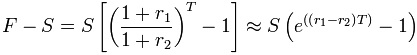F = forward rate

S = spot rate

r1 = simple interest rate of the term currency

r2 = simple interest rate of the base currency

T = tenor (calculated to the appropriate day count conversion)

2.      Future market

Margin account and margin call

CME (Chicago Merchandise Exchange)

 Product Code Contract Last Change Chart Open High Low Globex Vol 6EM6 JUN 2016 1.12775 -0.00025 1.12685 1.12880 1.12570 10,141 6JM6 JUN 2016 0.0089435 -0.0000175 0.0089555 0.0089640 0.0089335 23,494 6AM6 JUN 2016 0.7578 +0.0011 0.7551 0.7581 0.7540 10,392 6MM6 JUN 2016 0.057110 +0.000030 0.057000 0.057110 0.056960 1,385 6NM6 JUN 2016 0.6741 +0.0003 0.6741 0.6747 0.6731 2,141

# Euro FX Futures Contract Specs

 Contract Size 125,000 euro Contract Month Listings Twenty months in the March quarterly cycle (Mar, Jun, Sep, Dec) Settlement Procedure Physical Delivery Position Accountability 10,000 contracts Ticker Symbol CME Globex Electronic Markets: 6E Open Outcry (All-or-None only): EC AON Code: UG Minimum Price Increment \$.0001 per euro increments (\$12.50/contract). \$.00005 per euro increments (\$6.25/contract) for EUR/USD futures intra-currency spreads executed on the trading floor and electronically, and for AON transactions. Trading Hours CME Globex (ETH) Sundays: 5:00 p.m. – 4:00 p.m. Central Time (CT) next day. Monday – Friday: 5:00 p.m. – 4:00 p.m. CT the next day, except on Friday - closes at 4:00 p.m. and reopens Sunday at 5:00 p.m. CT. CME ClearPort Sunday – Friday 5:00 p.m. – 4:15 p.m. Chicago Time (CT) with a 45–minute break each day beginning at 4:15 p.m. Open Outcry (RTH) 7:20 a.m. – 2:00 p.m. Central Time (CT) Last Trade Date / Time View calendar 9:16 a.m. Central Time (CT) on the second business day immediately preceding the third Wednesday of the contract month (usually Monday). Exchange Rule These contracts are listed with, and subject to, the rules and regulations of CME. Block Trade Eligibility Yes. Block Trade Minimum 150 Contracts

# Euro FX Futures Prices Thr, Mar 20th, 2016

 Contract Last Change Open High Low Previous Volume Time Links 1.12488 +0.00087 1.12402 1.12589 1.12289 1.12401 0 00:18 1.12780 -0.00020 1.12685 1.12880 1.12570 1.12800 10,155 00:18 1.13170 -0.00015 1.13060 1.13175 1.13055 1.13185 9 03/21/16 1.13600s -0.00140 0.00000 1.13600 1.13600 1.13740 26 03/21/16 1.14020s -0.00145 1.14070 1.14070 1.13900 1.14165 26 03/21/16 1.14575s -0.00130 1.14490 1.14575 1.14490 1.14705 1 03/21/16 1.15095s -0.00115 1.14875 1.15095 1.14875 1.15210 1 03/21/16 1.15615s -0.00100 0.00000 1.15615 1.15615 1.15715 0 03/21/16 1.16135s -0.00090 0.00000 1.16135 1.16135 1.16225 0 03/21/16 1.16760s -0.00080 0.00000 1.16760 1.16760 1.16840 0 03/21/16 1.17390s -0.00065 0.00000 1.17390 1.17390 1.17455 0 03/21/16 1.18025s -0.00050 0.00000 1.18025 1.18025 1.18075 0 03/21/16 1.18655s -0.00035 0.00000 1.18655 1.18655 1.18690 0 03/21/16 1.19290s -0.00020 0.00000 1.19290 1.19290 1.19310 0 03/21/16 1.19920s -0.00010 0.00000 1.19920 1.19920 1.19930 0 03/21/16 1.20550s +0.00005 0.00000 1.20550 1.20550 1.20545 0 03/21/16

Euro Future Contract Specifications

 Symbol E6 Name Euro FX (6E) Exchange CME Trading Months March, June, September, December (H, M, U, Z) Trading Unit EUR 125,000 Tick Size 0.0001 points (\$12.50 per contract) – (minimum price movement) Daily Limit None Trading Hours 5:00p.m. - 4:00p.m. (Sun-Fri) CST Last Trading Day Second business day preceding third Wednesday of expiring month Value of one futures unit \$125,000 Value of one options unit \$125,000

Short and long position and payoff

For long position’s payoff: Value at maturity (short position) = principal * (spot-futures)

For short position’s payoff:  Value at maturity (short position) = -principal * (spot-futures)

Exercise 1: Amber sells a March futures contract and locks in the right to sell 500,000 Mexican pesos at \$0.10958/Ps (peso). If the spot exchange rate at maturity is \$0.095/Ps, the value of Amber’s position on settlement is? (refer to ppt)

Exercise 2: Amber sells a March futures contract and locks in the right to sell 500,000 Mexican pesos at \$0.10958/Ps (peso). If the spot exchange rate at maturity is \$0.11/Ps, the value of Amber’s position on settlement is? (refer to ppt)

Exercise 3: You expect peso to depreciate on 4/4. So you sell peso future contract (6/17) on 4/4 with future rate of \$0.09/peso. And on 6/17, the spot rate is \$0.08/peso. Calculate the value of your position on settlement (refer to ppt)

Exercise 4: Firm A have c\$500,000.00 payables due on 6/1. So it can purchase a future contract delivered on 6/1 to lock in the price to pay for Canadian dollars on 6/1. (refer to ppt)

Exercise 5: Firm A have c\$500,000.00 receivable due on 6/1. So it can sell a future contract delivered on 6/1 to lock in the price to sell the Canadian dollars on 6/1. (refer to ppt)

Currency Option market

NASDAQ OMX PHLX (Philadelphia Stock Exchange) trades more than 2,600 equity options, sector index options and U.S. dollar-settled options on major currencies. PHLX offers a combination of cutting-edge electronic and floor-based options trading.

Morning star:  http://quote.morningstar.com/Option/Options.aspx?Ticker=fxf

1.      What is Call and put option? Difference between the two?

2.      Calculate the payoff for both call and put?

·         For call: Profit = Spot rate – (strike price + premium)

Example: Jim is a speculator . He buys a British pound call option with a strike of \$1.4 and a December settlement date. Current spot price as of that date is \$1.39. He pays a premium of \$0.12 per unit for the call option. Just before the expiration date, the spot rate of the British pound is \$1.41.At that time, he exercises the call option and sells the pounds at the spot rate to a bank. One option contract specifies 31,250 units. What is Jim’s profit or loss? Assume Linda is the seller of the call option. What is Linda’s profit or loss? (refer to ppt)

·         For put: Profit = strike price – (spot rate + premium)

Example A speculator bought a put option (Put premium on £ = \$0.04 / unit, X=\$1.4, One contract specifies £31,250 )

He exercise the option shortly before expiration, when the spot rate of the pound was \$1.30. What is his profit? What is the profit of the seller? (refer to ppt)

3.      Draw the payoff graph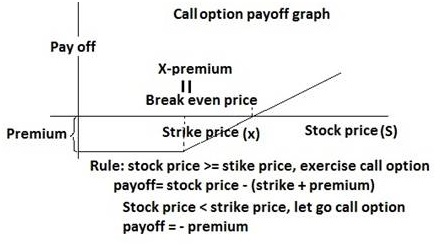HW of chapter 5:

1.                                          Consider a trader who opens a short futures position. The contract size is £62,500, the maturity is six months, and the initial price is \$1.50 = £1. The next day, the settlement price is \$1.60 = £1. What is the amount of his gain or loss?

2.                                          Consider a trader who opens a long futures position. The contract size is £62,500, the maturity is six months, and the initial price is \$1.50 = £1. The next day, the settlement price is \$1.60 = £1. What is the amount of his gain or loss?

3.                                          Consider a trader who opens a short futures position. The contract size is £62,500, the maturity is six months, and the initial price is \$1.50 = £1. The next day, the settlement price is \$1.40 = £1. What is the amount of his gain or loss?

4.                                          Consider a trader who opens a long futures position. The contract size is £62,500, the maturity is six months, and the initial price is \$1.50 = £1. The next day, the settlement price is \$1.40 = £1. What is the amount of his gain or loss?

5. You are a speculator who buy a put option on Swiss francs for a premium of \$.05, with an exercise price of \$.60. The option will not be exercised until the expiration date, if at all. If the spot rate of the Swiss franc is \$.55 on the expiration date, your net profit per unit, assuming that you have to buy Swiss francs in the market to fulfill your obligation, is?

6.   You purchase a call option on Swiss francs for a premium of \$.05, with an exercise price of \$.50. The option will not be exercised until the expiration date, if at all. If the spot rate on the expiration date is \$.58, your net profit per unit is?

7. You are a speculator who buy a call option on Swiss francs for a premium of \$.05, with an exercise price of \$.60. The option will not be exercised until the expiration date, if at all. If the spot rate of the Swiss franc is \$.55 on the expiration date, your net profit per unit, assuming that you have to buy Swiss francs in the market to fulfill your obligation, is?

8.   You purchase a put option on Swiss francs for a premium of \$.05, with an exercise price of \$.50. The option will not be exercised until the expiration date, if at all. If the spot rate on the expiration date is \$.58, your net profit per unit is?

# Million Dollar PipsThe Life Of A Day Trader

Foreign Exchange Market

# Futures - Mechanics of the Futures Market

Currency war explained – bear talk cartoon

Chapter 7  International Arbitrage And Interest Rate Parity

1.      Locational arbitrage

£ in \$:              \$1.60               \$1.61               \$1.62      \$1.63

How can you arbitrage? (Answer: Buy pound at bank1’s ask price and sell pound at bank2’s bid price. Profit is \$0.01/pound)

£ in \$:             \$1.6                 \$1.61               \$1.61      \$1.62

How can you arbitrage?(Answer: Buy pound at bank1’s ask price and sell pound at bank2’s bid price. No Profit )

Exercise 2: If you start with \$10,000 and conduct one round trip transaction, how many \$ will you end up with ?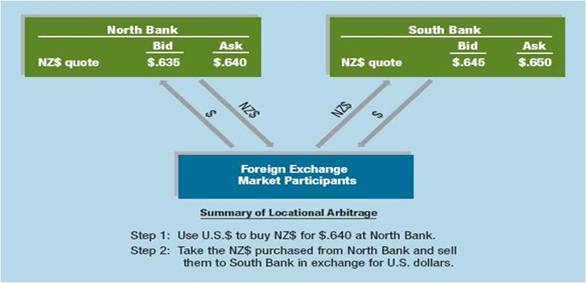(Answer: (\$10000 / 0.64(\$/NZ\$)) – the amount obtained from north bank.

(\$10000 / 0.64(\$/NZ\$))  * 0.645 (\$/NZ\$)  = \$10078.13)

2.      Triangular arbitrage

Exercise 1: £ is quoted at \$1.60. Malaysian Rinnggit (MYR) is quoted at \$0.20 and the cross exchange rate is £1 = MYR 8.1. How can you arbitrage? (answer: Either \$ è MYR è £ è \$, or \$ è £ è MYR è \$, one way or another, you should make money. In this case, it is the latter one. Imagine you have \$1.6 è 1 pound (£1 = MYR 8.1) è MYR8.1 è \$1.62(1MYR = 0.2\$, so 8.1 *0.2= 1.62\$) è profit of \$0.2 from an initial investment of \$1.6

Exercise 2: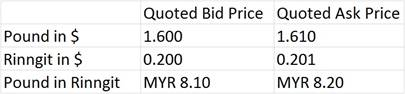How can you arbitrage with the above information?

\$1.61 è buy pound 1 pound è sell pound for rinngit @ 1 pound = 8.1 MYR; so get 8.1MYR è sell Rinngit for \$ @ bid price.  8.1MYR =  8.1 * 0.20 = \$1.62, a profit of \$0.01 out of \$1.61 initial investment)

3.      Covered Interest Arbitrage (CIA):

Exercise 1: Assume you have \$800,000 to invest. Current spot rate of pound is \$1.60. 90 day forward rate of pound is \$1.60. 90 day interest rate in US is 2%. 90 day interest rate in UK is 4%.  How can you arbitrage?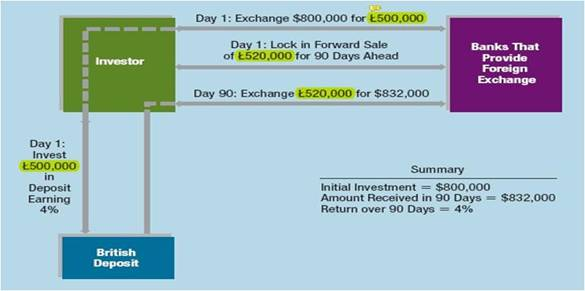(Answer: Convert at spot rate for pound and then deposit pound in UK bank. 90 days later, convert back to \$ at forward rate. Refer to the above graph for details)

Exercise 2:  You have \$100,000 to invest for one year. How can you benefit from engaging in CIA?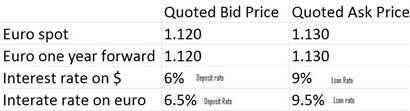(Answer: Again, buy at ask and sell at bid.  Convert at spot rate for pound and then deposit pound in UK bank. One year later, convert back to \$ at forward rate. (\$100,000 / 1.13)*(1+6.5%) *1.12 = \$105,558. However, if keep the money in US, you can get \$100,000*(1+6%) = \$106,000 So better to deposit in US and do not participate in CIA)

Interest rate parity (IRP)

·         The interest rate parity implies that the expected return on domestic assets = the exchanged rate adjusted expected return on foreign currency assets.

·          Investors cannot earn arbitrage profits by

1.      borrowing in a country with a lower interest rate

2.      exchanging for foreign currency

3.      and investing in a foreign country with a higher interest rate

4.      due to gains or losses from exchanging back to their domestic currency at maturity.

Equation of IRP: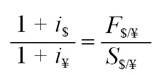or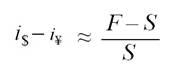Or,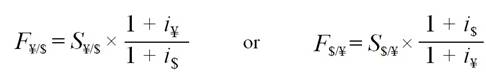Exercise 1: ih is 5%; if  is 6%; If the foreign currency’s spot rate is \$0.10. Then what is the foreign currency’s forward rate?

(Answer: Fh/f / Sh/f = (1+ ih)/(1+if) = (1+5%)/(1+6%)  S= \$0.1, so F=(1.05/1.06)*0.1 = \$0.099)

Exercise 2:  i\$ is 8%; iSF  is 4%;  If spot rate S = 1.48SF/\$, then how much is F90 (90 day forward rate)?

(Answer: F SF/\$ / SSF/\$ = (1+ iSF)/(1+i\$) = (1+4%/4)/(1+8%/4)  S=1.48, F=(1.01/1.02)*1.48 = 1.465SF/\$)

Exercise 3:  i\$ is 8%; iyen  is 4%;  If spot rate S = 106YEN/\$, then how much is F180 (180 day forward rate)?

(Answer: F YEN/\$ / SYEN/\$ = (1+ iYEN)/(1+i\$) = (1+4%/2)/(1+8%/2)  S=106, F=(1.02/1.04)*106 = 103.96 yen/\$)

Exercise 4: i\$ is 4% and i£ is 2%. S is \$1.5/£ and F is \$2/£. Does IRP hold? How can you arbitrage? What is the forward rate in equilibrium?

(Answer: F\$/£ / S\$/£= (1+ i\$)/(1+i£) = (1+4%)/(1+2%)  S= 1.5, so F=(1.04/1.02)*1.5 = \$1.529/£, so F is too high and should sell forward contract not buy it. So UK residents can make profits from CIA and US residents should deposit in US)

Exercise 5:  i\$  is 2% and  i£  is 4%. S is \$1.5/£ and F is \$1.1/£. Does IRP hold? How can you arbitrage? What is the forward rate in equilibrium?

(Answer: F\$/£ / S\$/£= (1+ i\$)/(1+i£) = (1+2%)/(1+4%)  S= 1.5, so F=(1.02/1.04)*1.5 = \$1.471/£, so F is too low and should buy forward contract. So US residents should arbitrage)

Rule of Thumb:

·         All that is required to make a covered interest arbitrage profit is for interest rate parity not to hold.

·         The key to determining whether to start CIA is to compare the differences in interest rate to the forward premium.

Arbitrage rule of thumb:

·         If the difference in interest rates is greater than the forward premium, invest in the higher interest yielding currency.

·         If the difference in interest rates is less than the forward premium, invest in the lower interest yielding currency.

 Spot exchange rate S(\$/£) = \$2.0000/£ 360-day forward rate F360(\$/£) = \$2.0100/£ U.S. discount rate i\$ = 3.00% British discount rate i£ = 2.5%

1.       With above information and \$1,000 in hand, any opportunities?

2.      When  F360(\$/£) = \$2.50/£?

3.      When  F360(\$/£) = \$1.90/£

(Answer: 1. Either CIA make 3% or deposit in US also 3%. F is priced correctly.

2.      F is too high for US residents and should keep money in US.

3.      F is too low and arbitrage opportunity. )

The followings are useful websites

Exchange rate forecast

Daily FX News(has news, technical analysis and live rates):http://www.dailyfx.com/

Technical analysis _ chart example book

Forex Trend lines

Historical currency rate

Historical currency chart

Chapter 8 Purchasing Power Parity, International Fisher Effect

1)      The Law of one price THEORY:

All else being equal (no transaction costs), a product’s price should be the same in all markets

So price in \$ sold in US = price in \$ sold in Japan after conversion to \$ from ¥

P\$  = P ¥ * Spot Rate \$/¥

Where the price of the product in US dollars (P\$), multiplied by the spot exchange rate (S,  dollar per yen), equals the price of the product in Japanese yen (P¥)

Or,  S =  P\$/   P ¥

PPP states that the spot exchange rate is determined by the relative prices of similar basket of goods.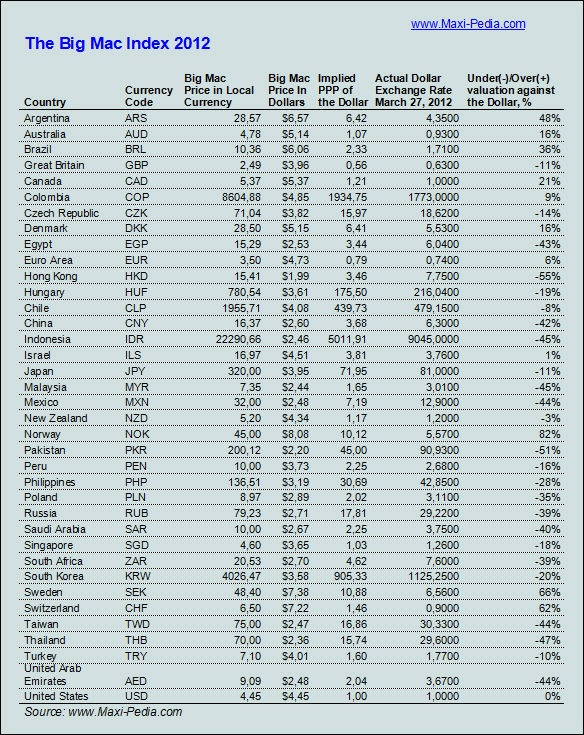Question: calculate the exchange rate of China, Canada, Russia, Turkey, Egypt, and New Zealand based on the above big mac index. Find the actual exchange rate and explain why there is a gap.

3)      Relative purchasing power parity: Calculate changes in exchange rate based on inflation in two countries

◦      the relative change in prices between countries over a period of time determines the change in exchange rates

◦      if the spot rate between 2 countries starts in equilibrium, any change in the differential rate of inflation between them tends to be offset over the long run by an equal but opposite change in the spot rate

Example 1: 1£=1.6\$. US inflation rate is 9%. UK inflation is 5%. What will happen? Calculate the new exchange rate using the following equation.

(US inflation is 4% higher than UK  US products are 4% higher than UK  US customers convert \$ to £ to purchase cheap UK products This buying pressuring of £ and selling pressure of \$  will force £ to appreciate  until the prices in UK are the same as in US àNo benefits for US customers to buy from UK market.)

Math equation: ef= Ih- If  or ((1+ Ih)/(1+If) -1= ef)

Answer: (1+ 9%) /(1+5%) -1 =  ef = 4% , and 1£=1.6\$, so the new rate of £ =1.6*(1+4%) = 1.66 £/\$.

Example 2: 1£=1.6\$. US inflation rate is 5%. UK inflation is 9%. What will happen? Calculate the new exchange rate using the PPP equation.

Answer: ef = Ih If, Ih= 5%, If =9%, so ef = 5%-9% = -4%, so the old rate is that 1£=1.6\$. The new rate should be 4% lower. So new rate is that  1£=1.6*(1-4%) = 1.536\$

Example 3: 1£=1.2. Inflation rate in Germany is 4%. UK inflation is 9%. What will happen? Calculate the new exchange rate using the PPP equation.

Answer:  Home currency is euro and foreign currency is pound. ef = Ih If, Ih= 4%, If =9%, so ef = 4%-9% = -5%, so the old rate is that 1£=1.2. The new rate should be 5% lower. So new rate is that  1£=1.2*(1-5%) = 1.14

4)      International Fisher Effect

Fisher Effect: Nominal interest rate (R) = real interest rate (r) + inflation (I)

By assuming real interest rates in two countries are the same, we conclude that inflation moves along with the nominal interest rate which is observable and reported.

International fisher effect: ef= Rh- Rf  or  ((1+ Rh)/(1+Rf) -1= ef)

Example 4: If the interest rate of US is 10% and that of UK is 5%,  which countrys currency will appreciate, by how much? Imagine 1£=1.6\$.

Answer:  Home currency is \$ and foreign currency is . ef = Rh Rf, Rh= 10%, Rf =5%, so ef = 10%-5% = 5%, so the old rate is that 1£=1.6\$. The new rate should be 5% higher. So new rate is that  1£=1.6*(1+5%) = 1.68\$

Example 5: If the interest rate of US is 5% and that of UK is 10%, which country’s currency will appreciate, by how much? Imagine 1£=1.6\$.

Answer:  Home currency is \$ and foreign currency is £. ef = Rh Rf, Rh= 10%, Rf =5%, so ef = 10%-5% = 5%, so the old rate is that 1£=1.6\$. The new rate should be 5% higher. So new rate is that  1£=1.6*(1+5%) = 1.68\$

Chapter 7 and 8 Homework

1. If a Big Mac costs \$2 in the United States and 300 yen in Japan, what is the estimated exchange rate of yen/ \$ as hypothesized by the Big Mac index?

1. Interest rates are currently 2% in the US and 3% in Germany.  The current spot rate between the € and \$ is \$1.5/€. What is the expected spot rate in one year if the international Fisher effect holds?

3.      Suppose that the one-year interest rate is 5.0 percent in the United States and 3.5 percent in Germany, and the one-year forward exchange rate is \$1.3/€. What must the spot exchange rate be?

4.      Imagine that can borrow either \$1,000,000 or €800,000 for one year. The one-year interest rate in the U.S. is i\$ = 2% and in the euro zone the one-year interest rate is i = 6%. The one-year forward exchange rate is \$1.20 = €1.00; what must the spot rate be to eliminate arbitrage opportunities?

1. Today’s spot exchange rate is €1.00 = \$1.25 and the rates of inflation expected to prevail for the next year in the US = 2% and 3% in the euro zone. What is the one year forward rate?n market.

1. If a Big Mac costs \$2 in the United States and 300 yen in Japan, what is the estimated exchange rate of yen/ \$ as hypothesized by the Big Mac index?

1. Interest rates are currently 2% in the US and 3% in Germany.  The current spot rate between the € and \$ is \$1.5/€. What is the expected spot rate in one year if the international Fisher effect holds?

8.      Suppose that the one-year interest rate is 5.0 percent in the United States and 3.5 percent in Germany, and the one-year forward exchange rate is \$1.3/€. What must the spot exchange rate be?

9.      Imagine that can borrow either \$1,000,000 or €800,000 for one year. The one-year interest rate in the U.S. is i\$ = 2% and in the euro zone the one-year interest rate is i = 6%. The one-year forward exchange rate is \$1.20 = €1.00; what must the spot rate be to eliminate arbitrage opportunities?

10.  Image that the future contracts with a value of  €10,000 are available. The information of one year interest rates, spot rate and forward rate available are as follows.

Question: profits that you can make with one contract at maturity?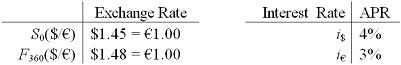11.              Image that you find that interest rate per year is 3% in Italy. You also realize that the spot rate is \$1.2/€ and forward rate (one year maturity) is \$1.18/€.

Question: Use IRP to calculate the interest rate per year in US.

12.  You observed the following quotes.

Spot rate of Singapore dollar in \$= \$.32 / Singapore dollar

Spot rate of  £ in U.S. \$= \$1.50 /£

Spot rate of £ in Singapore dollars = S\$4.50 / £

Question: Use triangular arbitrage, figure out a strategy to make profits. And how much is your profit?

13.  You observed the following bid and ask rates of £ from two local banks as follows:

Bank C               \$1.61                  \$1.63

Bank D               \$1.58                  \$1.60

Question: Use locational arbitrage approach to figure out a profitable strategy. And how much is your profit?

14.  You find that inflation in Japan just reduced to 1.3%, while in US, the inflation rate just increased to 3%. You also observed that the spot rate for yen was \$0.0075 before the adjustment by economists. With new inflation released, the demand and supply for currencies will drive the exchange rate to a new equilibrium price.

Question: Use PPP to estimate the new exchange rate for yen.

15.              You observed the nominal interest rate (annual) just increased to 7% in China, while the nominal annual interest rate is 5% in US. The spot rate for Chinese Yuan is \$6.6 before the adjustment.

Question: Use IFE to estimate the new spot rate for Chinese Yuan after the interest rate changes.

Subject: Managing Transaction Exposure

Chapter 11: Transaction Exposure

2.      Types of foreign exchange exposure

5.        Transaction Exposure measures changes in the value of outstanding financial obligations incurred prior to a change in exchange rates but not to be settled until after the exchange rate changes

6.        Operating (Economic)Exposure – also called economic exposure, measures the change in the present value of the firm resulting from any change in expected future operating cash flows caused by an unexpected change in exchange rates

Translation Exposure – also called accounting exposure, is the potential for accounting derived changes in owner’s equity to occur because of the need to “translate” financial statements of foreign subsidiaries into a single reporting currency for consolidated financial statements

Tax Exposure – the tax consequence of foreign exchange exposure varies by country, however as a general rule only realized foreign losses are deductible for purposes of calculating income taxes

\

What is transaction exposure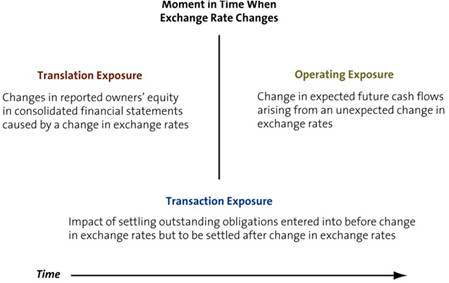Example of transaction exposure

Purchasing or selling on credit goods or services when prices are stated in foreign currencies

Borrowing or lending funds when repayment is to be made in a foreign currency

Being a party to an unperformed forward contract and

Otherwise acquiring assets or incurring liabilities denominated in foreign currencies

How to reduce the transaction exposure risk?

1.      1. Forward (Future) Market Hedge

2.      2. Money Market Hedge

3.      3. Options Market Hedge: call and put

·         To hedge a foreign currency payable buy calls on the currency.

·         To hedge a foreign currency receivable buy puts on the currency.

Exercise 1:  Hedging currency payable (refer to the PPT for answers)

A U.S.–based importer of Italian bicycles

·         In one year owes €100,000 to an Italian supplier.

·         The spot exchange rate is \$1.18 = €1.00

·         The one year forward rate is \$1.20 = €1.00

·         The one-year interest rate in Italy is i = 5%

·         The one-year interest rate in US is i\$ = 8%

Call option exercise price is \$1.2/ € with premium of \$0.03.

How to hedge the currency payable risk

a.       With forward contract?

b.      With money market?

c.       With call option? Can we use put option?

Answer: Need €100,000 one year from now to pay the payable and plan to hedge the risk of overpaying for the payable one year from now.

1)      With forward contract:

Buy the one year forward contract @\$1.20 = €1.00. So need 100,000€*1.2\$/€ = \$120,000 one year from now.

2)      With money market:

Need €100,000one year from now, and the rate is 5% in Italy, so can deposit €100,000/(1+5%) = €95238.10 now.

For this purpose, need to convert €95238.10*\$1.18 /€=\$112380.98.

Imagine the company does not have that much of cash and it borrows @8%. So one year from now, the total required to pay back to the banks is: \$112380.98 *(1+8%) = \$121371.43.

Summary: Borrow \$112380.98 @8% and convert to €95238.10 at present; One year later, the company can get the €100,000 and needs to pay back to the bank a total of \$121371.43.

3)      With call option:

Imagine the rate one year later is \$1.25/€. So should exercise the call option and the cost one year later should be

€100,000 *(1.2+0.03) \$/€ = \$123000, lower than the actual cost without the call option.

Exercise 2:  Hedging currency receivable (refer to the PPT for answers)

·         A U.S.–based exporter of US bicycles to Swiss distributors

·         In 6 months receive SF200,000 from an Swiss distributor

·         The spot exchange rate is \$0.71 = SF1.00

·         The 6 month forward rate is \$0.71 = SF1.00

·         The one-year interest rate in Swiss is iSF = 5%

·         The one-year interest rate in US is i\$ = 8%

·         Put option exercise price is \$0.72/ SF with premium of \$0.02.

How to hedge the currency payable risk

a.       With forward contract?

b.      With money market?

c.       With call option? Can we use put option?

Answer: Will receive SF200000 six month from now as receivable and plan to hedge the risk of losing value in the receivable six month from now.

1)      With forward contract:

Sell the one year forward contract @\$0.71 = €1.00. So get 200,000SF * 0.71\$/SF = \$142,000 six month from now.

2)      With money market:

Get SF200000 six month from now, and the rate is 5% in Swiss (or 2.5% for six months), so can borrow SF 200,000/(1+2.5%) = SF195121.95 now.

And can convert @ spot rate to SF195121.95 * 0.71\$/SF = \$138536.59. This is the money you have now.

So six month from now, the total you have in the bank is: \$138536.59*(1+4%) = \$144078.05. And you can use the SF200000 receivable to pay back the loan.

Summary: Borrow SF195121.95 @5% at present; six month later, the company can get the SF200,000 receivable and payback the loan. Meanwhile, convert the borrowed SF to \$ and deposit in US banks @ 8%.

3)      With put option: With SF200000 received six month later, need to converting it back to \$. So can buy put option which allows to sell SF for \$ at the exercise price \$0.72/ SF.

Imagine the rate one year later is \$0.66/ SF. So should exercise the put option and the  total amount of \$ six month later should be SF 200,000 *(0.72-0.02) \$/ SF = \$140000.

Homework:

1.      Suppose that your company will be billed £10 million payable in one year.  The money market interest rates and foreign exchange rates are given as follows. How to hedge the risk for parable using forward contract. How to hedge the risk using money market? How to hedge risk using call option?

 Call option exercise price The U.S. one-year interest rate: \$1.46/ € with  premium of \$0.03 6.10% per annum The U.K. one-year interest rate: 9.00% per annum The spot exchange rate: \$1.50/£ The one-year forward exchange rate \$1.46/£

2.      Suppose that your company will be billed £10 million receivable in one year.  The money market interest rates and foreign exchange rates are given as follows. How to hedge the risk for parable using forward contract. How to hedge the risk using money market? How to hedge risk using put option?

 put option exercise price The U.S. one-year interest rate: \$1.46/ € with  premium of \$0.03 6.10% per annum The U.K. one-year interest rate: 9.00% per annum The spot exchange rate: \$1.50/£ The one-year forward exchange rate \$1.46/£

Interest rate swap

Swaps lead to cash flow exchanges on several future dates

·         Unlike a forward rate agreement or currency forward, which leads to the exchange of cash flows on just one future date

Interest rate swap:  two parties will agree to: term, fixed rate, floating rate benchmark (commonly LIBOR), notional principal, and payment frequency.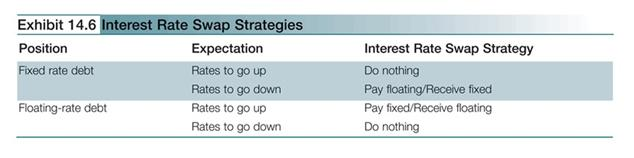Plain vanilla swap: An agreement between two parties to exchange fixed-rate for floating-rate financial obligations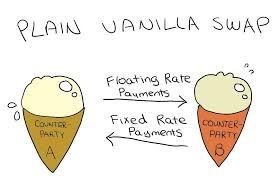Exercise: Party A has a debt obligation with a floating interest rate of LIBOR+1.5%; Party B has a debt obligation with a fixed rate of 7.3%. Party A anticipates of future increases in interest rate; Party B just sees differently. How can the two parties hedge again their foreseeable risk in interest rate?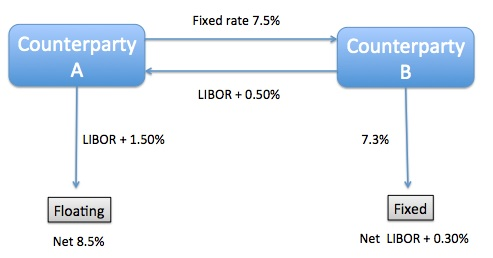Party A is paying floating rate on its obligation, but wants to pay fixed rate.  Party B is paying fixed rate, but wants to pay floating rate. They can enter into an interest rate swap, and the net result will be that each party can ‘swap’ their existing obligation for their desired obligation.

NO HW requirement

Final (in the 8th week on 4/26 at 6pm)

Review session: Monday from 2-4pm and Tuesday from 2-4pm

Final Exam Study Guide

Part I - Multiple choices and True / False (22 questions, and 2 points each)

1.   Iterest rate swap concept.
2. Iterest rate swap concept.

3. Plain vanilla swap concept

5.   The international Fisher effect (IFE) concept

6.   The international Fisher effect (IFE) concept

7.   Conceptof interest rate parity, locational arbitrage, and purchasing power parity.

8.   How to hedge receivables?

9.   How to hedge receivables?

10. How to hedge payable?

11. How to hedge payable?

12. How to hedge payable?

13. How to hedge using put call option?

14. How to hedge using put call option?

15. Interest rate parity concept

16. Interest rate parity concept

18. How to hedge payable?

19. How to hedge payable?

22. Interest rate parity concept

Part II  - Short Answer Questions: (total 56 points)

1: use IRP formula: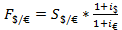to get forward rate

2: Use PPP, given spot rate inflation rates in both countries, calculate new spot rate.

3:  Simiar to exercise in class (Chapter 11), what is the hedged value if using a forward market hedge?  What is the hedged value if using a money market hedge?

4: Simiar to exercise in class (Chapter 11), how to hedge using forward, money market and option market?

5.  Forward contract calculation given spot rate, forward rate.

6. Put call option calculation

7. Put call option calculation

8. Forward contract calculation given spot rate, forward rate.

9. Consider a trader who opens a short futures position. Calculate the profit earned

10. Consider a trader who opens a long futures position.  Calculate the profit earned